# NCERT Solutions Class 12 Maths Chapter 3 MATRIX

Ncert Solutions for class 12 subject Maths Chapter 3 MATRIXin pdf Best Free NCERT Solutions for class 1 to 12 in pdf NCERT Solutions, cbse board, Maths, ncert Solutions for Class 12 Maths, class 12 Maths ncert solutions, MATRIX, Class 12, ncert solutions chapter 3 MATRIX, class 12 Maths, class 12 Maths ncert solutions, Maths ncert solutions class 12, Ncert Solutions Class 12 Mathematics Chapter 3 MATRIX

## Exercise 3.1 Chapter 3 Class 12 Maths MATRIX Ncert Solutions

Question 1. In the matrix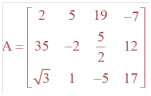, write
(i) The order of the matrix, (ii) The number of elements,
(iii) Write the elements a13, a21, a33, a24, a23.

Question 2. If a matrix has 24 elements, what are the possible orders it can have? What, if it has 13 elements?

Question 3. If a matrix has 18 elements, what are the possible orders it can have? What, if it has 5 elements ?

Question 4. Construct a 2 × 2 matrix, A = [aij], whose elements are given by:
(i)aij=(i + j)2/2

Question 4. Construct a 2 × 2 matrix, A = [aij], whose elements are given by:
(ii)aij=i/j

Question 4. Construct a 2 × 2 matrix, A = [aij], whose elements are given by:
(iii)aij=(i + 2j)2/2

Question 5. Construct a 3 × 4 matrix, whose elements are given by:
(i)aij=1/2| -3i + j |

Question 5. Construct a 3 × 4 matrix, whose elements are given by:
(ii)aij=2i - j

Question 6. Find the values of x, y and z from the following equations:
(i)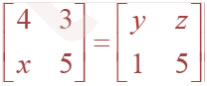Question 6. Find the values of x, y and z from the following equations:
(ii)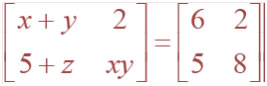Question 6. Find the values of x, y and z from the following equations:
(iii)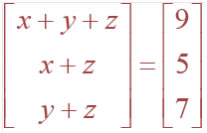Question 7. Find the value of a, b, c and d from the equation: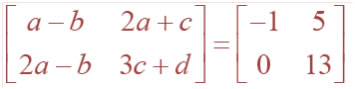Question 8.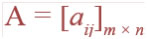is a square matrix, if
(A) m < n (B) m > n (C) m = n (D) None of these

Question 9.Which of the given values of x and y make the following pair of matrices equal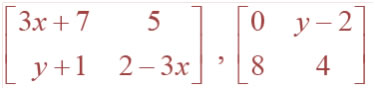Question 10. The number of all possible matrices of order 3 × 3 with each entry 0 or 1 is:

## Exercise 3.2 Chapter 3 Class 12 Maths MATRIX Ncert Solutions

Question 1. Let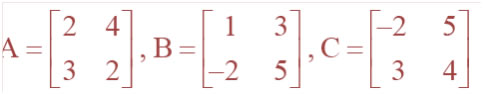Find each of the following:
(i) A + B (ii) A – B (iii) 3A – C

Question 1. LetFind each of the following:
(iv) AB (v) BA

Question 2. Compute the following:

# (iii) and (iv)

Question 3. Compute the indicated products.

Question 4. If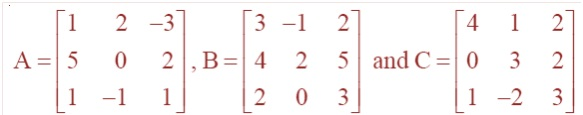, then compute (A+B) and (B – C). Also, verify that A + (B – C) = (A + B) – C.

Question 5. If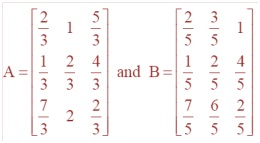, then compute 3A – 5B.

Question 6. Simplify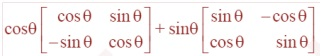### NCERT Books Free Pdf Download for Class 5, 6, 7, 8, 9, 10 , 11, 12 Hindi and English Medium

 Mathematics Biology Psychology Chemistry English Economics Sociology Hindi Business Studies Geography Science Political Science Statistics Physics Accountancy